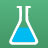cancel
Showing results for
Did you mean:New Contributor

## How long does 700ml of acetone take to boil completely away at 56 C?

I have 700ml of acetone in a 1000ml flask in a heating mantle, as part of a fractional distillation set up. I am wondering what the rate of boiling is so I can set a timer and stop burning the impurities in my acetone by leaving it on the heat for too long. I know I can just time it myself but I am curious about the math too.New Contributor

## Re: How long does 700ml of acetone take to boil completely away at 56 C?

The short answer to this is that the rate that it will boil away will depend on the amount of heat per second that you are putting into it. The hotter the heating mantle is, the faster it will boil. You need to know the heat output of the heating mantle to determine this.

However, if you know the power output of the heating mantle some quick math can tell you the time it will take :

Since acetone has a heat of vaporization of 31.3 kJ/mol at its normal boiling point of 56 Celcius, a density of 784 kg/m^3, and a molar mass of 58.08 g/mol, then the required heat to evaporate 1 mL would be:

(31.3x10^3 J/mol) / (58.08 g/mol) x (784 kg/m^3) x (1000 g/kg) / (100^3 cm^3/m^3) x (1 mL/cm^3) = 422 J/mL

Meaning you would need about 296 kJ to evaporate all 700 mL of it. Divide this by the power output of the heating mantle (in Watts) and you'll have your answer.

Hope this helps!